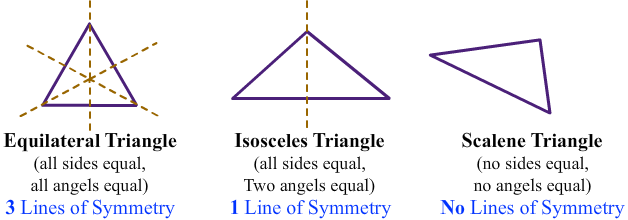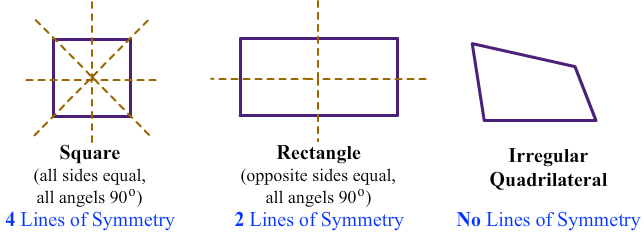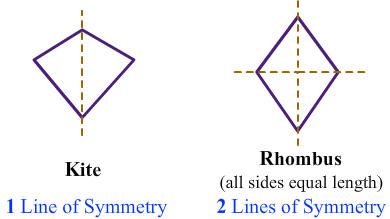# Lines of Symmetry

Print Rate 0 stars Common Core
Lesson size:
Message preview:
Someone you know has shared lesson with you:

To play this lesson, click on the link below:

https://www.turtlediary.com/lesson/lines-of-symmetry.html

Hope you have a good experience with this site and recommend to your friends too.

Login to rate activities and track progress.
Login to rate activities and track progress.

Line of symmetry is a line that divides a figure into two congruent parts, each of which is the mirror image of the other.

When the figure having a line of symmetry is folded along the line of symmetry, the two parts should coincide.For example, the dotted line divides the butterfly into two halves that are mirror image of each other. This dotted line is line of symmetry.

These two sides are reflections or mirror images, and is also referred to as reflection symmetry.

Let's identify the line of symmetry of some common polygons.

Triangles

A triangle can have 3, or 1 or no line of symmetry.Different types of quadrilaterals have different number of lines of symmetry.## Lines of Symmetry

• Line of symmetry is a line that divides a figure into two congruent parts, each of which is the mirror image of the other.
• When the figure having a line of symmetry is folded along the line of symmetry, the two parts should coincide.
• Different polygons have different number of lines of symmetry.

## Similar Lessons

Become premium member to get unlimited access.